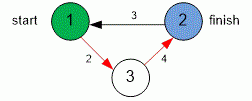favorite We need a little bit of your help to keep things running, click on this banner to learn more
Competitions

# Dijkstra

The directed weighted graph is given. Find the shortest distance from one vertex to another.

#### Input

The first line contains three integers n, s and f (1n2000; 1s, fn), where n is the number of graph vertices, s is the initial vertex, and f is the final vertex. Each of the next n lines contains n integers - the matrix of weights of the graph, where -1 means the lack of edge between the vertices, and any nonnegative integer - the weight of the existing edge. The main diagonal of the matrix contains zeroes.

#### Output

Print the shortest distance from s to f or -1 if the path does not exist.Time limit 2 seconds
Memory limit 128 MiB
Input example #1
```3 1 2
0 -1 2
3 0 -1
-1 4 0
```
Output example #1
```6
```